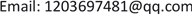1. 引言

2. 一类确定性金融系统的参数分析2.1. 一类金融系统的改进

{ x ˙ = z + ( y − a ) x y ˙ = 1 − b y − x 2 z ˙ = − x − c z (1)

{ x ˙ = r z + ( y − a ) x + k x y ˙ = 1 − b y − x 2 z ˙ = − x − c z (2)

2.2. 确定性系统局部稳定性与分岔

E 1 = ( 0 , 1 b , 0 )

E 2 = ( 1 − b r c − a b + k b , r c + a − k , − 1 c 1 − b r c − a b + k b )

E 3 = ( − 1 − b r c − a b + k b , r c + a − k , 1 c 1 − b r c − a b + k b )

2.2.1. 平衡点E<sub>1</sub>的pitchfork分岔与Hopf分岔

F 1 ( λ ) = ( λ + b ) [ ( 1 b − a + k − λ ) ( c + λ ) − r ] = 0

2.2.2. 平衡点E<sub>2</sub>和E<sub>3</sub>的Hopf分岔

F 2 , 3 ( λ ) = λ 3 + p λ 2 + q λ + s = 0

q = 2 k b + b c + 2 − 3 b r c − 2 a b

s = 2 k b c + 2 c − 2 b r − 2 a b c

B = 2 a b c − 3 b 2 c − 2 c − 2 k b c 3 b

C = 2 b c 2 + b 2 c 3 + b c 4 − 2 a b 2 c 2 + 2 k b 2 c 2 3 b

3. 随机平衡点的灵敏度

{ x ˙ = r z + ( y − a ) x + k x + ε w ˙ 1 y ˙ = 1 − b y − x 2 + ε w ˙ 2 z ˙ = − x − c z + ε w ˙ 3 (3)

x ˙ = f ( x ) d t + ε σ w ˙ (4)

x 是一个3维向量， w ( t ) 是一个3维维纳过程， ε 是强度参数。

σ = [ 1 0 0 0 1 0 0 0 1 ]

v ( x ) = − lim ε → 0 ε 2 log ρ ( x , ε )

ρ t ( x , ε ) ≈ ρ t ∗ ( x , ε ) = N ∗ exp ( − ( x − x ¯ ) T W − 1 ( x − x ¯ ) 2 ε 2 )

A W + W A T + S = 0 (5)

W = [ 445.4985 − 165.9164 − 393.8766 − 165.9164 153.3293 166.0713 − 393.8766 166.0713 354.9389 ]

4. 随机系统的稳定控制

x ˙ = f ( x , u ) + ε σ w ˙ (6)

d z = A z + ε σ w ˙

d z = ( A + B K ) z d t + ε σ w ˙

( A + B K ) W + W ( A + B K ) T + S = 0 (7)

M 是随机灵敏度矩阵的集合，其中元素满足对称和正定性。

Φ = { K | Re λ i ( A + B K ) < 1 } , λ i ( A ) 是矩阵A的特征值。

P 2 H ( ( W ) P 2 = 0 (8)

K = K ¯ + C , K ¯ = B + H ( W ) ( 1 2 P 1 − I ) W − 1 (9)

C是满足 B C W + W C T B T = 0 任意的 1 × 3 矩阵。

5. 随机受控金融系统的数值模拟

{ x ˙ = r z + ( y − a ) x + k x + u + ε w ˙ 1 y ˙ = 1 − b y − x 2 + ε w ˙ 2 z ˙ = − x − c z + ε w ˙ 3 (10)

E ( w i ( t ) − w i ( s ) ) = 0 , E ( w i ( t ) − w i ( s ) ) 2 = | t − s | ， u 是控制部分。由图3可以看出在 [ 0.5 , 0.55 ] 和 [ 0.9 , 0.99 ] 之间，平衡点的随机灵敏度非常大。

A = [ y ¯ − a + k x ¯ r − 2 x ¯ − b 0 − 1 0 − c ] , B = [ 1 0 0 ] ,

K = [ k 1 k 2 k 3 ] , S = [ 1 0 0 0 1 0 0 0 1 ] ,

P 1 = [ 1 0 0 0 0 0 0 0 0 ] , P 2 = [ 0 0 0 0 1 0 0 0 1 ] ,

W = [ w 11 w 12 w 13 w 12 w 22 w 23 w 13 w 23 w 33 ] .

{ − 4 x ¯ w 12 − 2 b w 22 + 1 = 0 − 2 w 13 − 2 c w 33 + 1 = 0 − 2 x ¯ w 13 − w 12 − ( b + c ) w 23 = 0

w 12 = − 0.4 x ¯ , w 22 = 1 + 1.6 x ¯ 2 2 b , w 33 = 3 10 c

W = [ 1 − 0.4 x ¯ 0.2 − 0.4 x ¯ 1 + 1.6 x ¯ 2 2 b 0 0.2 0 3 10 c ]

6. 结论

1) 不加控制项的随机系统的随机轨迹会发散在平衡位置周围，加入控制项后，可以将随机轨迹稳定在平衡位置的小邻域内。

2) 加入控制项后可以实现随机振荡的稳定，并且其稳定性的实现是由于受控系统平衡位置的随机灵敏度的降低。